# ARM > Introduction to ARM > Barrel Shifter

by on

## Barrel Shifter

The barrel shifter is a functional unit which can be used in a number of different circumstances. It provides five types of shifts and rotates which can be applied to Operand2. (These are not operations themselves in ARM mode.)

## `LSL` – Logical Shift Left

Example: Logical Shift Left by 4.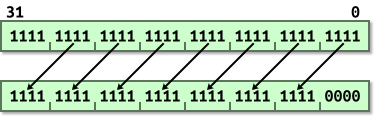Equivalent to `<<` in C.

## `LSR` – Logical Shift Right

Example: Logical Shift Right by 4.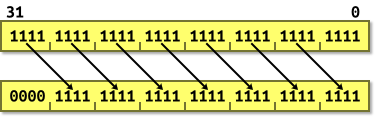Equivalent to `>>` in C. i.e. unsigned division by a power of 2.

## `ASR` – Arithmetic Shift Right

Example: Arithmetic Shift Right by 4, positive value.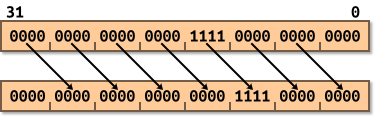Example: Arithmetic Shift Right by 4, negative value.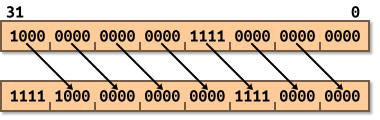Equivalent to `>>` in C. i.e. signed division by a power of 2.

## `ROR` – Rotate Right

Example: Rotate Right by 4.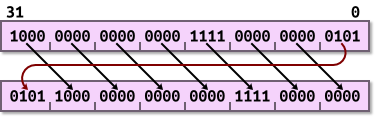Bit rotate with wrap-around.

## `RRX` – Rotate Right Extended

Example: Rotate Right Extended.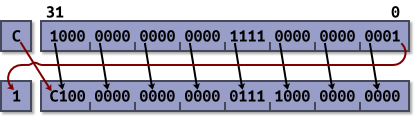33-bit rotate with wrap-around through carry bit.

## Examples of Barrel Shifting

• `MOV r0, r0, LSL #1`
• Multiply R0 by two.
• `MOV r1, r1, LSR #2`
• Divide R1 by four (unsigned).
• `MOV r2, r2, ASR #2`
• Divide R2 by four (signed).
• `MOV r3, r3, ROR #16`
• Swap the top and bottom halves of R3.
• `ADD r4, r4, r4, LSL #4`
• Multiply R4 by 17. (N = N + N * 16)
• `RSB r5, r5, r5, LSL #5`
• Multiply R5 by 31. (N = N * 32 - N)

## Remarks

At the start I mentioned that certain C operations don’t map onto available CPU operations. `ROR` and `RRX` are two of those operations.

Certain ARM instructions such as `MUL`, `CLZ` and `QADD` cannot use the barrel shifter.

Note that right shifting negative signed quantities is strictly implementation defined behaviour in C. The compiler is allowed to choose whether it performs a logical or an arithmetic shift. (That said, it’s always implemented as an arithmetic shift in all of the ARM toolchains the author has used.)